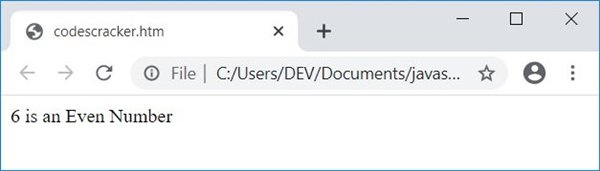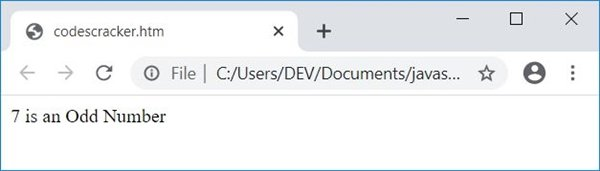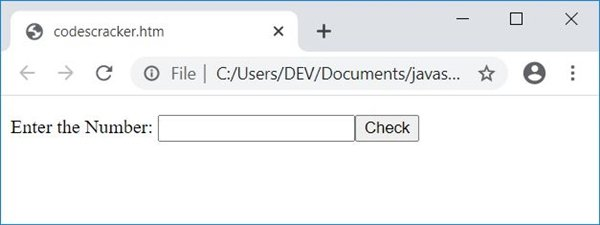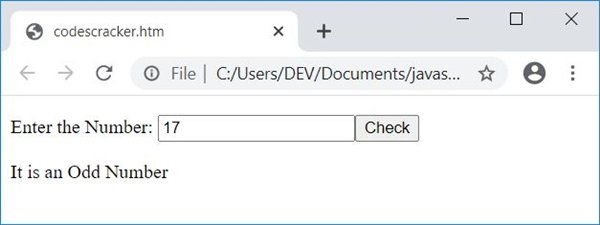# JavaScript Program to Check an Even or Odd Number

In this article, you will learn how to check whether a number is an even or an odd number with and without using user input in JavaScript. Here is the list of JavaScript programs to check even or odd numbers:

• Check even or odd without user input.
• Receive a number from the user, check, and print whether the number is even or odd. Live output of this program is also provided.

## Check even or odd numbers in JavaScript

This program checks whether the value stored in the num variable is an even or an odd number. The document.write() method writes the data to an HTML output.

```<!doctype html>
<html>
<body>
<script>
var num=6;
if(num%2==0)
document.write(num + " is an Even Number");
else
document.write(num + " is an Odd Number");
</script>
</body>
</html>```

Save this code in a file with .html extension. Now open the file in a web browser. Here is the output:If you change the value of num to 7 and save the code in the same file, then here is the output after refreshing or re-opening the file in a web browser:### Allow the user to enter the number

This is the actual program to check whether a number entered by the user is an even or an odd number in JavaScript. This program receives input from the user using a text box.

```<!doctype html>
<html>
<script>
var num, temp;
function fun()
{
num = parseInt(document.getElementById("num").value);
if(num)
{
temp = document.getElementById("resPara");
temp.style.display = "block";
if(num%2==0)
document.getElementById("res").innerHTML = "Even";
else
document.getElementById("res").innerHTML = "Odd";
}
}
</script>
<body>

<p>Enter the Number: <input id="num"><button onclick="fun()">Check</button></p>
<p id="resPara" style="display:none;">It is an <span id="res"></span> Number</p>

</body>
</html>```

Here is its sample output:Now enter any number, say 17, and click on the "Check" button to print a message that tells whether the entered number is an even or an odd number, as shown in the snapshot of the final output given below:The following code:

`style="display:none;"`

is a CSS code that hides an HTML element where it is written. Because it is written inside a P (paragraph) tag, whose id is resPara. So this paragraph gets hidden initially.

When the user clicks the "Check" button, a function named fun() gets called. All the statements in this function get executed. The following JavaScript statement:

`num = parseInt(document.getElementById("num").value);`

states that the int (integer) value of an HTML element whose id is num gets initialized to the num variable. And the statement given below:

`temp.style.display = "block";`

states that an HTML element whose id is stored in temp gets visible after executing this JavaScript statement. And the following JavaScript statement:

`document.getElementById("res").innerHTML = "Even";`

states that the text Even gets written to an HTML element whose id is res.

#### Live Output of the Previous Program

Here is the live output produced by the previous JavaScript program on checking an even or odd number:

Enter the Number:

JavaScript Online Test

« JavaScript Tutorial CodesCracker »